Multivibrator

Multivibrators are frequently used electronic circuits. They are capable of switching back and forth between two states. This makes them useful for a large variety of applications, as diverse as blinking signal systems and signal delays. However, multivibrators are probably most widespread in digital electronics in the form of flip-flops. In this tutorial you will get to know the basics of multivibrators as well as some practical applications on the breadboard.

Astable Multivibrator

The Astable Multivibrator is a very simple oscillator circuit that can be used to generate square waves.

It consists of only two NPN transistors, two capacitors, and four resistors, organized in a symmetric fashion. The collectors of the transistors are outputs, which provide complement square wave signals. The values of the capacitors and resistors can varied depending on the required frequency, and their values do not have to be symmetric.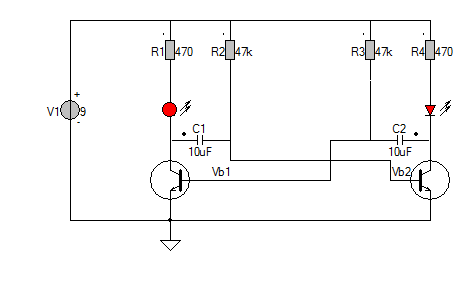How does the circuit work?

To begin, when power is applied, theoretically both T1 and T2 should turn on, since their base pins are connected through resistors (R2 and R3) to the plus battery voltage. However, due to small differences in the electric properties, one of the them will turn on slightly earlier than the other. Without loss of generality, let�s assume T2 turns on first. Therefore, T2�s collector begins to conduct and can be thought of as �shorted� to ground. Note that C2�s right lead is connected to T2�s collector, and since C2 is not charged yet, its left lead also has a voltage close to ground. This immediately shuts off T1, i.e. T1 becomes an open circuit. Therefore C1�s left lead is in floating status, and its right lead is connected to T2�s base, which is about 0.7V due to the forward voltage drop of transistors. This forward voltage drop is the same as a diode. So at this moment, the circuit is equivalent to the following:During this period, the collector of the right NPN remains low, and C2 begins to charge through R3. Thus the voltage on C2�s left lead will rise, and the rising time depends on R3 x C2. At the same time, C1 also charges, through R1, which is typically a small resistor (e.g. 100-1000 ohm). So C1�s left lead will quickly rise up to the battery voltage and remains high.

As C2 continues to charge, a critical moment will happen when its left lead rise up to 0.7V, at which moment transistor T1 will turn on, and its collector will conduct to ground. Note that since C1�s left lead is connected to T1�s collector, it will also drop to ground voltage. As C1 is fully charged, it�s right lead will suddenly drop to a negative voltage (Minus-battery voltage). This will shut off T2 firmly. Therefore the circuit will suddenly transition to the following equivalent: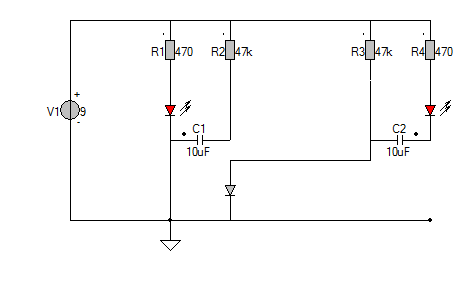During this period, the left NPN will remain conducting, and the collector of the right NPN will quickly rise to the positive battery voltage (due to the charging of C2 through a small resistor R4). At the same time, C1 charges through R2 and the voltage on its right lead will rise over time determined by R2 x C1. Now you can predict what�s going to happen: as C1 continues to charge, the next critical moment happens when C1�s right lead rises above 0.7V. At that point, T2 will conduct again while T1 shuts off. The same cycle will repeat.

The frequency of the oscillator is roughly $F=\frac{1}{0.693(R_2 C1 + R_3 C2)}$

With the given values in the first schematic (C1=C2=10uF, R2=R3=47K), the frequency is roughly 1.1Hz. These symmetric values can produce a square wave of 50% duty cycle. But they do not have to be symmetric. By using different R2 and R3 (or different C1 and C2), you can easily create waves with any desired duty cycle.

Let�s blink some LEDs with the oscillator. You can either connect LEDs in series with R1 and R4, or connect to the collectors of the transistors. Selecting 470Ω for the R1 and R4 limits the current through the LED's.

Also the signal on the base of the transistors are displayed. The result is on the left. As you can see, the beginning of stage 2 is signaled by the jump to a large negative value. During this stage, C1 charges through R2, so T2�s base voltage rises continuously. As soon as it crosses Vb, T2 conducts, and its base remains at Vb until the start of the next cycle.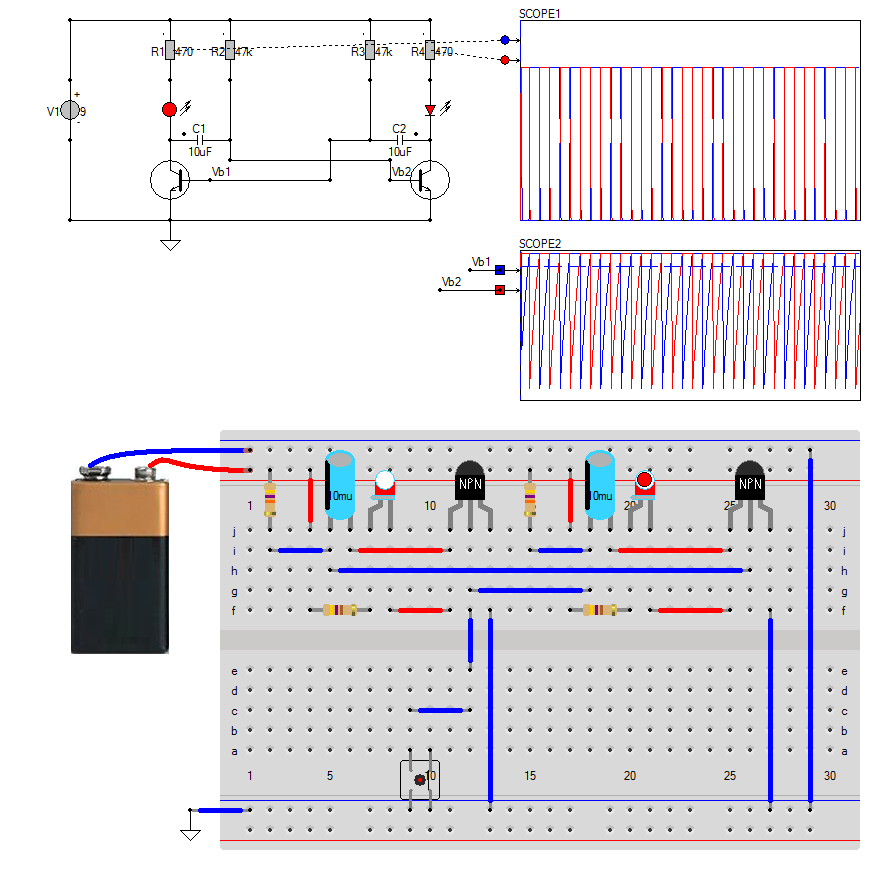There is small problem when dong a simulation of a Astable Multivibrator and that is starting. As mentioned before the autostart is because the transistors are a little bit different. In simulation they are the same, so everything remains at zero volt.

In the simulation the oscillation is started becuase there is a small leakage current (through the switch on the breadboard), or if there is an initial voltage on one of the capacitors(C1 has an initial voltage in hte simulation of the schematic).

Bistable Multivibrator

As the name indicates, a bistable multivibrator has 2 stable states. It can be switched from one state to another by providing an external trigger. It can be used for storing a binary digit (bit) as it has 2 stable states (0 or 1). So it is commonly used in digital circuits, where it is named as Flip Flop or Latches.

A bistable multivibrator can be easily constructed using the most popular and low cost 555 Timer IC.In the above circuit 555 is wired as a bistable multivibrator. Two switches are provided to SET(right switch button) or RESET(left switch button) the output of 555 and two LEDs are used for indicating the output. Resistors R3 & R4 are the Pull-Down(R3) Pull-Up(R4) resistors for the switches. Clicking the left switch is makes pin 6 high and will set the NE555.During the SET, pin 2 is pulled high by R4 so there is no conflicting reset signal. When the right switch closes, pin 2 will be in LOW state (pin 6 is already LOW) and the output of the NE555 will go to LOW state. Resistor R1 and R2 are the current liming resistors connected in series with the LED. Capacitor C1 is commonly connected to 5th pin of 555 for avoiding high frequency noises.

Keep the simulation running continuously by disabling the [Wait after screen] option in the simulation parameter dialog box, or simply disable it by clicking the buttonoffAstable Multivibrator

An Astable Multivibrator is an oscillator circuit that continuously produces rectangular wave without the aid of external triggering. The Astable Multivibrator is also known as Free Running Multivibrator. The Astable Multivibrator using a NE555 Timer is very simple, easy to design, very stable and low cost. It can be used for timing from microseconds to hours. Due to these reasons the NE555 has a large number of applications and it is a popular IC.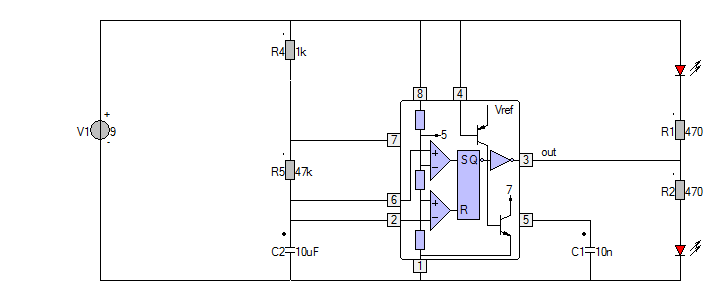The figure above shows the circuit diagram of the NE555 Timer wired in Astable Mode. Pin 8 and 1 of the IC are used for providing power. Pin 4 is a RESET pin which is active low and is connected to the battery plus voltage to avoid accidental resets. Pin 5 is the Control Voltage pin which is not used. So to avoid high frequency noises it is connected to a capacitor C1 (mostly 10nF) whose other end is connected to ground. Usually C1 = 0.01μF. The Trigger (pin 2) and Threshold (pin 6) inputs are connected to the capacitor C2 which determines the output of the timer. Pin 7, being the discharge pin is connected to the resistor R5 such that the capacitor can discharge through R5. A diode D connected in parallel to R5 can be used when a duty cycle less than or equal to 50% is required. The diode is not used here. The internal block diagram is shown in the schematic.Since the Control Voltage (pin 5) is not used the comparator reference voltages will be $$\frac{2}{3}V_{Battery}$$ and $$\frac{1}{3}V_{Battery}$$ respectively. So the output of the NE555 will set (goes high) when the capacitor voltage goes below $$\frac{1}{3}V_{Battery}$$ and output will reset (goes low) when the capacitor voltage goes above $$\frac{2}{3}V_{Battery}$$.

Principle of operation

• When the circuit is switched ON, the capacitor (C) voltage will be less than $$\frac{1}{3}V_{Battery}$$. So the output of the lower comparator will be HIGH and of the higher comparator will be LOW. This SETs the output of the SR Flip-flop.
• Thus the discharging transistor will be OFF and the capacitor C starts charging from Vcc through resistor Ra & Rb.
• When the capacitor voltage will become greater than $$\frac{1}{3}V_{Battery}$$ ( less than $$\frac{2}{3}V_{Battery}$$ ), the output of both comparators will be LOW and the output of SR Flip-flop will be same as the previous condition. Thus the capacitor continuous to charge.
• When the capacitor voltage will becomes slightly greater than $$\frac{2}{3}V_{Battery}$$ the output of the higher comparator will be HIGH and of lower comparator will be LOW. This resets the SR Flip-flop.
• Thus the discharging transistor turns ON and the capacitor starts discharging through resistor Rb.
• Soon the capacitor voltage will be less than $$\frac{2}{3}V_{Battery}$$ and output of both comparators will be LOW. So the output of the SR Flip-flop will be the previous state.
• So the discharging of capacitor continuous.
• When the capacitor voltage will become less than $$\frac{1}{3}V_{Battery}$$, the output SETs since the output of lower comparator is HIGH and of higher comparator is LOW and the capacitor starts charging again.
• This process continuous and a rectangular wave will be obtained at the output.

Principle of design

Capacitor Charges through R4 and R5. $T_{high} = 0.693(R4 + R5)C2$

Capacitor Discharges through R5 $T_{low} = 0.693 \cdot R5 \cdot C2$

Output Frequency $F_s = \frac{1}{T_{low} + T_{high}} = \frac{1.44}{(R4 + 2 R5) * C2}$

Duty Cycle $d = \frac{T_{high}}{T_{high} + T_{low}}$

Where $$T_{high}$$ and $$T_{low}$$ are the time period of HIGH and LOW of the output of the NE555.

From this we can find that Duty Cycle less than or equal to 50% cannot be obtained. There are two ways to obtain this.

1. Inverting the output
2. Using a Diode Parallel to resistor R5

Using a Diode Parallel to resistor R5

In this method, just compliment the output. Thus the Duty Cycle equals $d = \frac{T_{low}}{T_{high} + T_{low}}$Where $$T_{low}$$ and $$T_{high}$$ are the time periods of LOW and HIGH of the output of the NE555. In this method the duty cycle of the output of the NE555 will be greater than 50% and that of inverted output of the NE555 will be less than 50%. In this method we will connect a diode parallel to resistor R5 facing downwards. The charging current of capacitor C2 will bypass the resistor R5. Thus a Duty Cycle less than or equal to 50% can easily be obtained.
$$d_{NE555} = 1 - d_{Inverted-NE555}$$ $T_{high} = 0.693 \cdot R4 \cdot C2$

The simulation below shows the Astable Multivibrator. Do not forget to turn Animation on, so you can see the LEDs blinking.Clock Signal

A clock signal for logic circuits can be build using a NE555. The red trace in the scope shows the charging and discharging voltage over the capacitor. When reaching $$\frac{2}{3}V_{Battery}$$ and $$\frac{1}{3}V_{Battery}$$ the output changes state.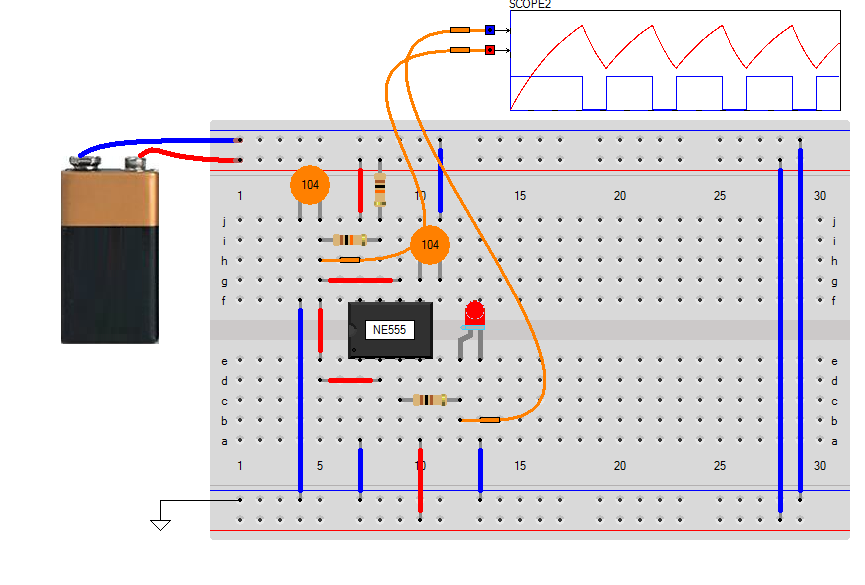Calculator

Seconds
%
�F

Click the button to calculate the new values for R4 and R5